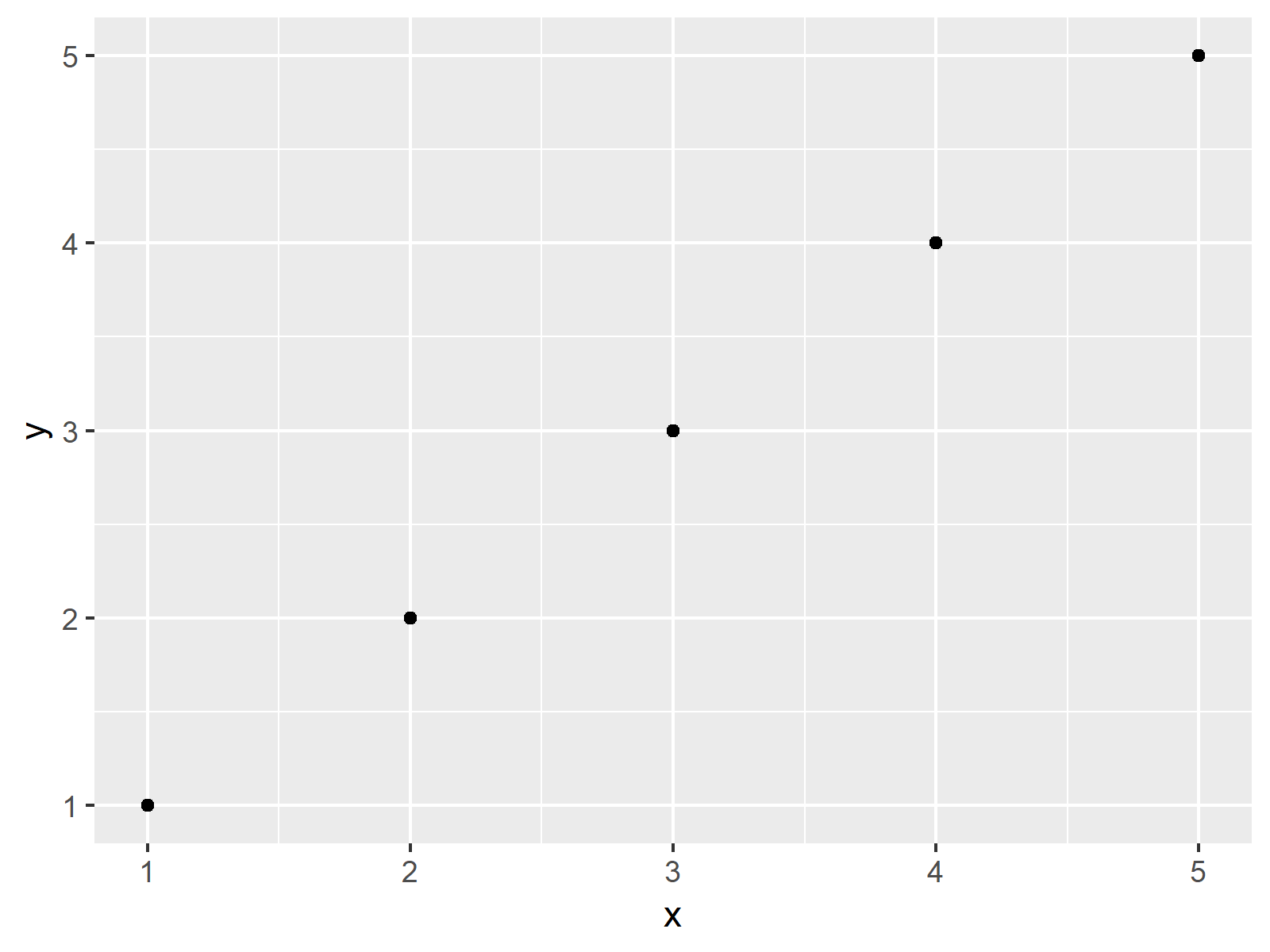# ggplot2 Plot in Script is not Displayed in R (Example)

This tutorial shows how to show a ggplot2 graphic when running a script in the R programming language.

The tutorial consists of the following content blocks:

So without further additions, let’s do this!

## Example Data, Add-On Packages & Default Plot

As a first step, let’s construct some exemplifying data:

```data <- data.frame(x = 1:5,        # Example data
y = 1:5)
data                               # Show example data
#   x y
# 1 1 1
# 2 2 2
# 3 3 3
# 4 4 4
# 5 5 5```

The previously shown output of the RStudio console shows that our example data has five rows and two numeric columns.

If we want to use the functions & commands of the ggplot2 package, we also have to install and load ggplot2:

```install.packages("ggplot2")          # Install ggplot2 package

Next, we may try to plot our data as shown below:

```for(i in 1:3) {                    # for-loop creating ggplot2 plot

if(i == 2) {

ggp <- ggplot(data, aes(x, y)) +
geom_point()
ggp
}
}```

Surprise! Nothing happened! So why didn’t our ggplot2 plot show up? That’s what I’m going to explain next…

## Example: Drawing ggplot2 Plot within for-Loop Using print Function

The following code shows how to return a ggplot2 plot within a long R programming script. If we want to draw a plot within a loop or a user-defined function, we can simply use the print() function to show our plot.

Run the following R syntax:

```for(i in 1:3) {                    # for-loop creating ggplot2 plot

if(i == 2) {

ggp <- ggplot(data, aes(x, y)) +
geom_point()
print(ggp)                     # Explicit printing of plot
}
}```The output of the previous syntax is shown in Figure 1 – A ggplot2 scatterplot drawn within a for-loop with if-condition.

## Video, Further Resources & Summary

Would you like to learn more about the ggplot2 package and its variety of functions, then please check out the video below, where you get a detailed introduction to the package and data visualization in R.

Please accept YouTube cookies to play this video. By accepting you will be accessing content from YouTube, a service provided by an external third party.If you accept this notice, your choice will be saved and the page will refresh.

Besides that, you could read the related tutorials of this website:

Summary: You learned in this article how to display ggplot2 plots within a running script in R. If you have additional comments and/or questions, don’t hesitate to let me know in the comments section.

Subscribe to the Statistics Globe Newsletter